# RD Sharma Solutions for Class 8 Maths Chapter - 7 Factorization Exercise 7.8

In Exercise 7.8 Chapter 7 Factorization of RD Sharma, we shall discuss the polynomials and factorization of quadratic polynomials of a theorem. Expert tutors at BYJU’S have designed solutions in order to help students who wish to learn short cut methods and simple techniques to solve difficult problems. Practising RD Sharma Solutions textbook is the best way to secure good marks in their board exams. Download free pdf of RD Sharma Solutions from the links provided below.

## Download the pdf of RD Sharma Solutions For Class 8 Maths Exercise 7.8 Chapter 7 Factorization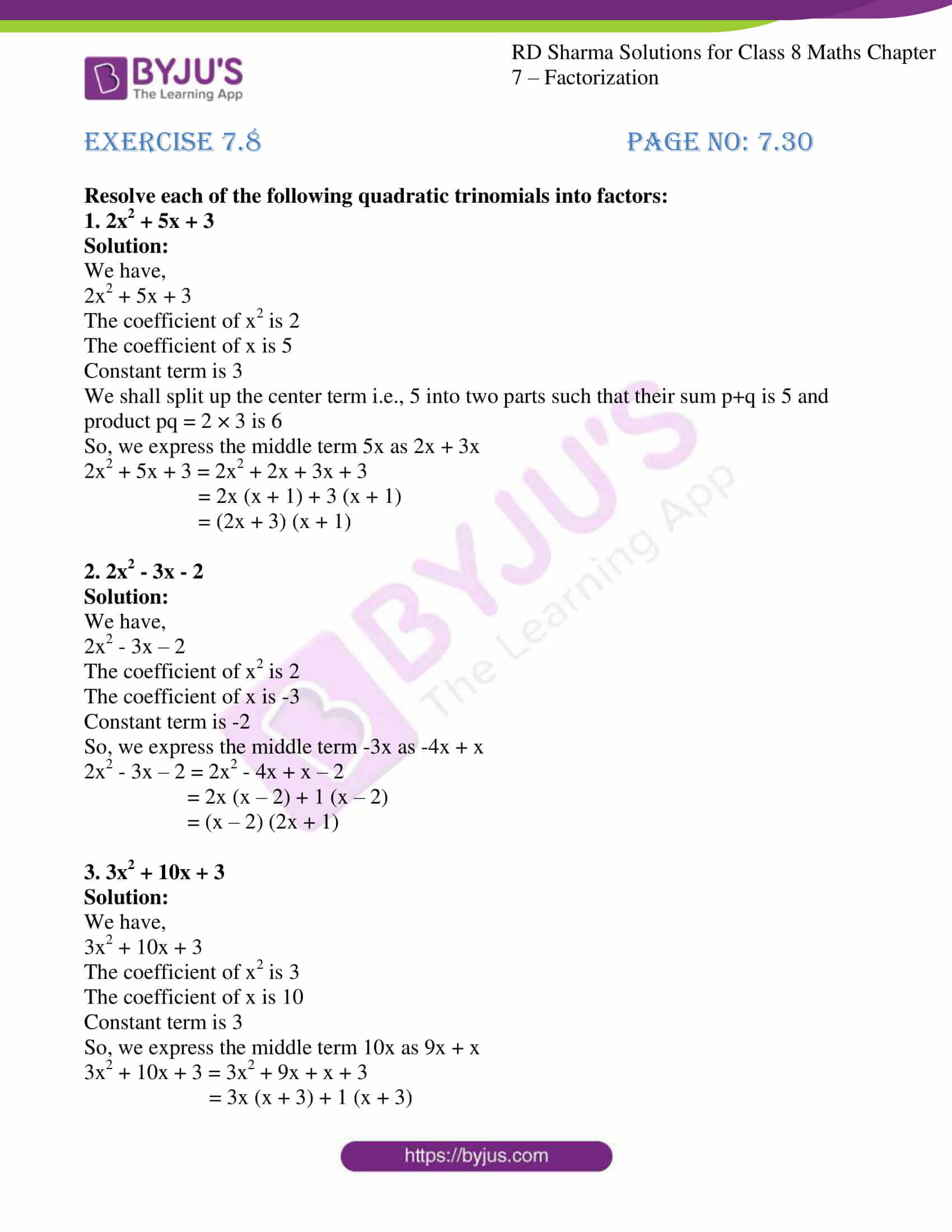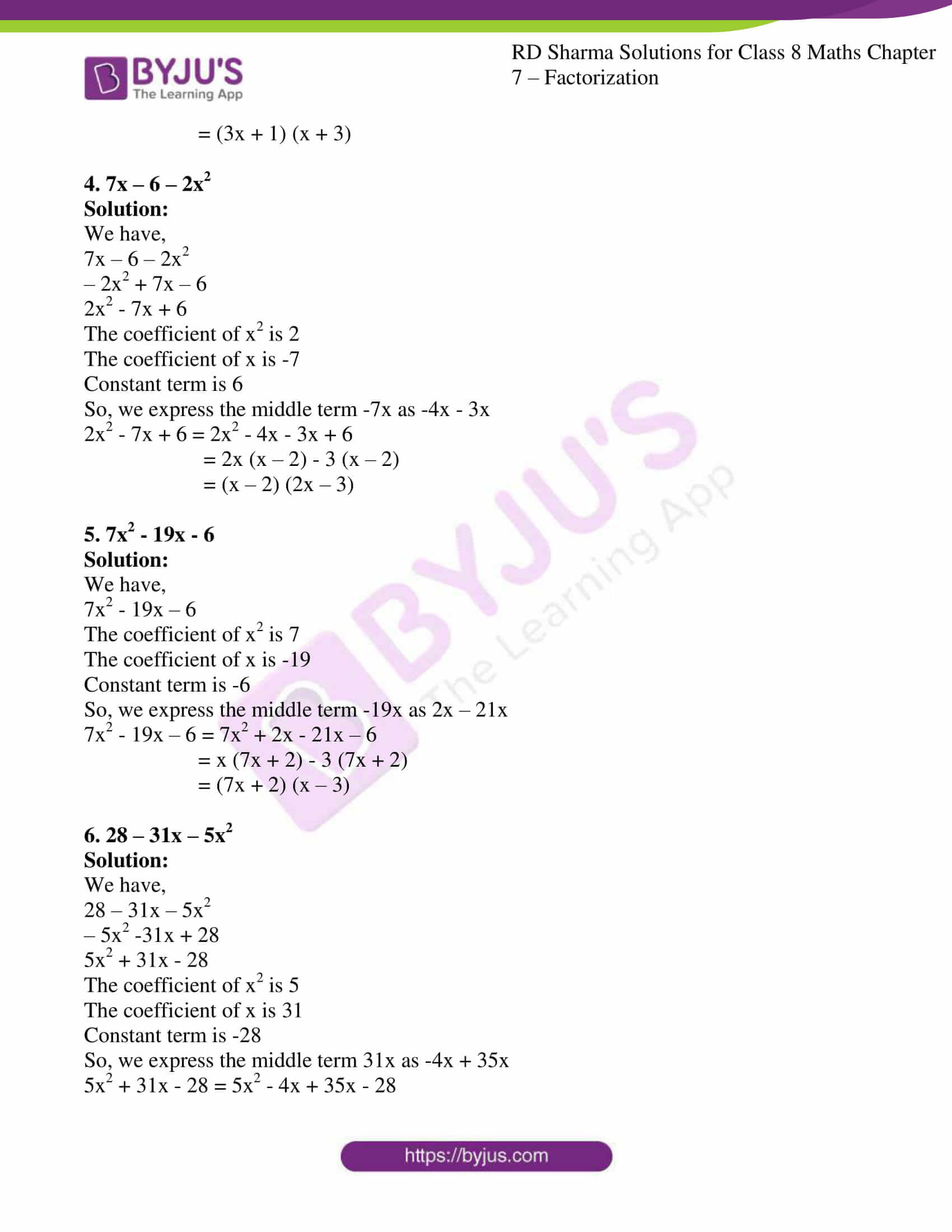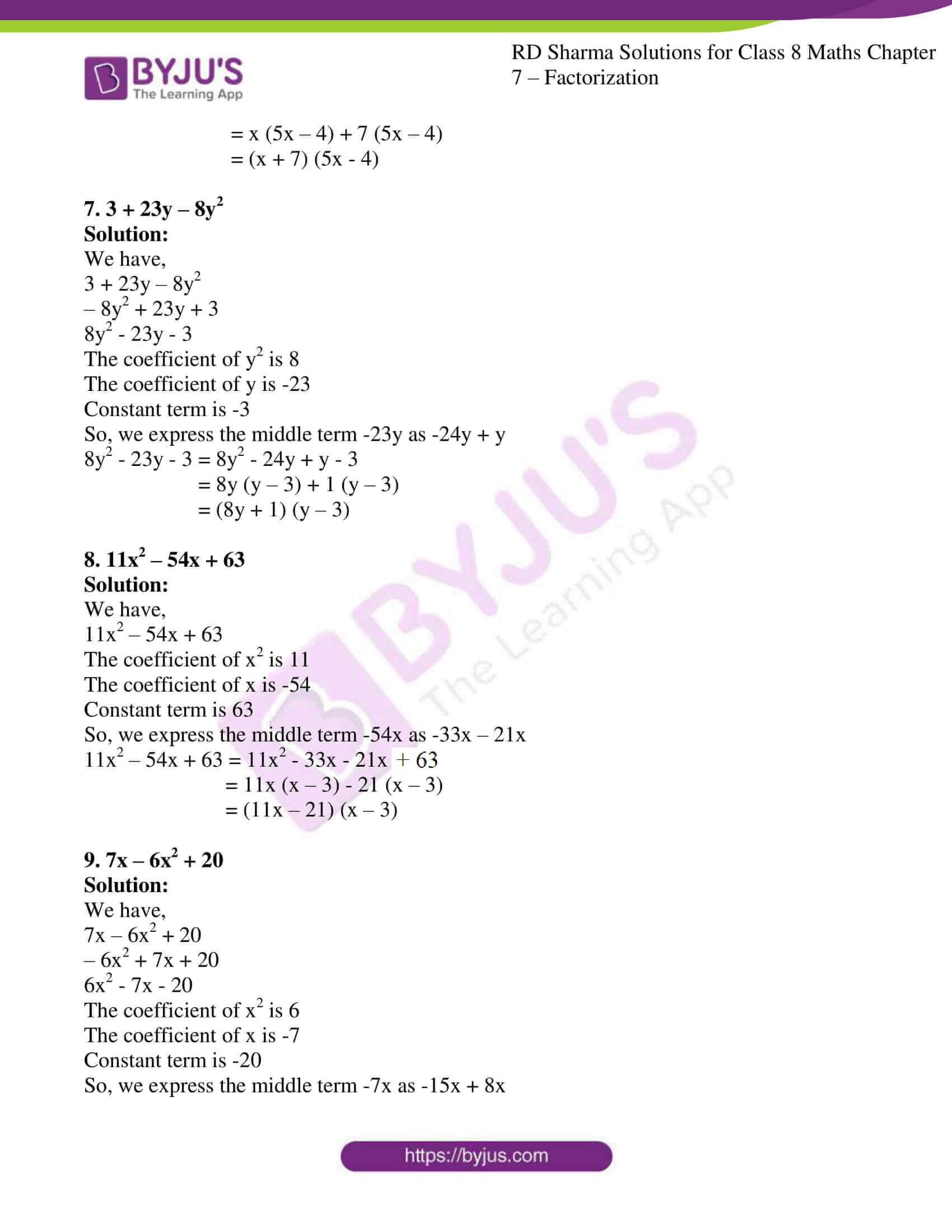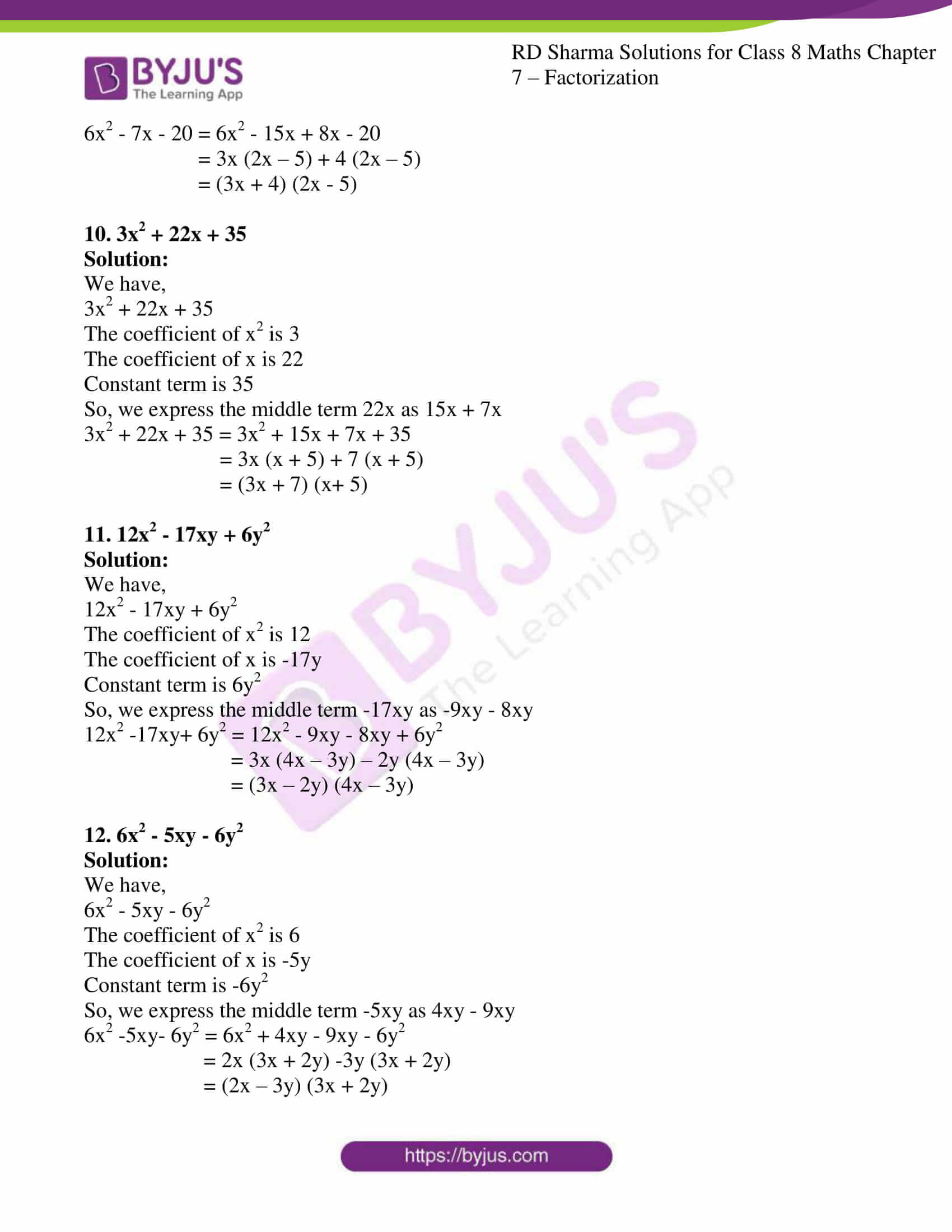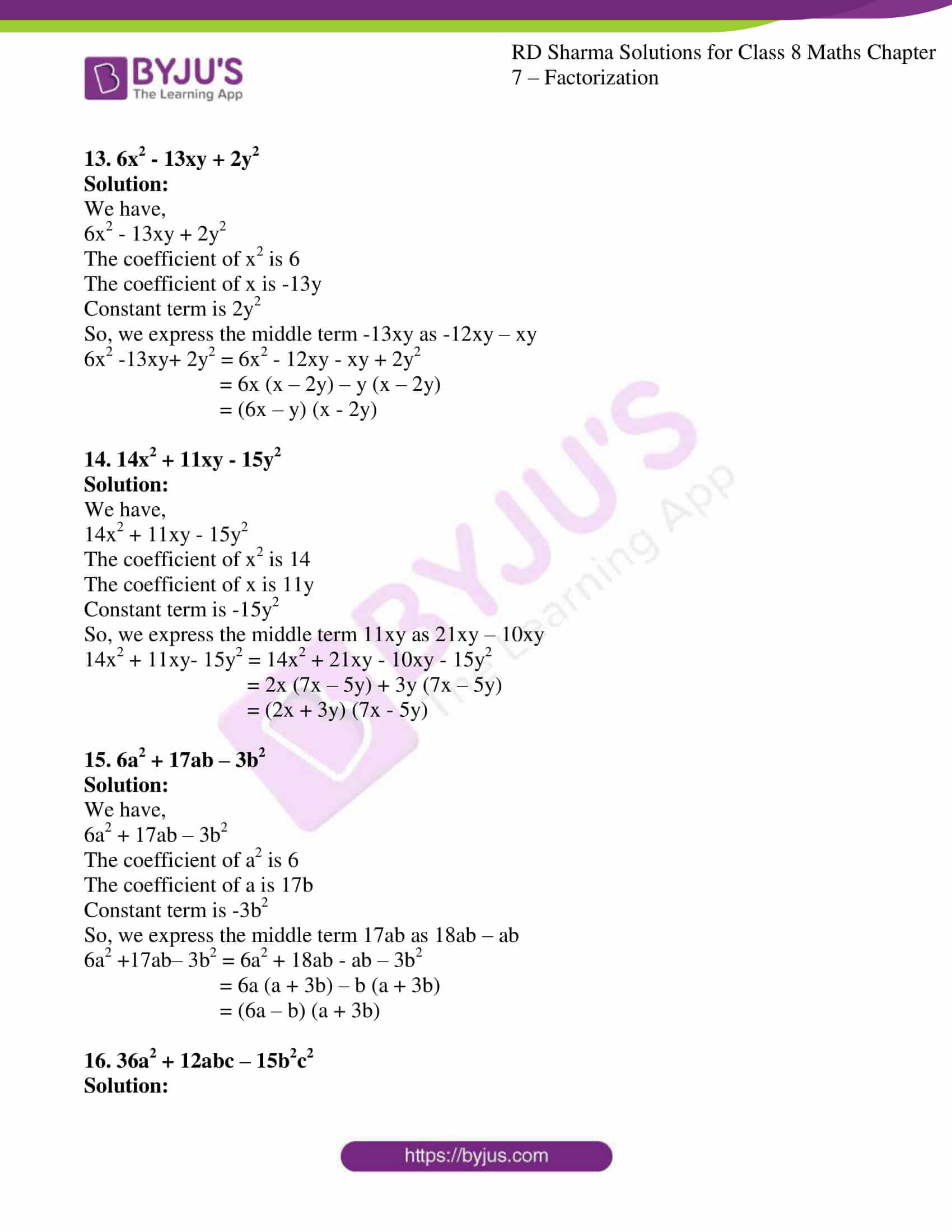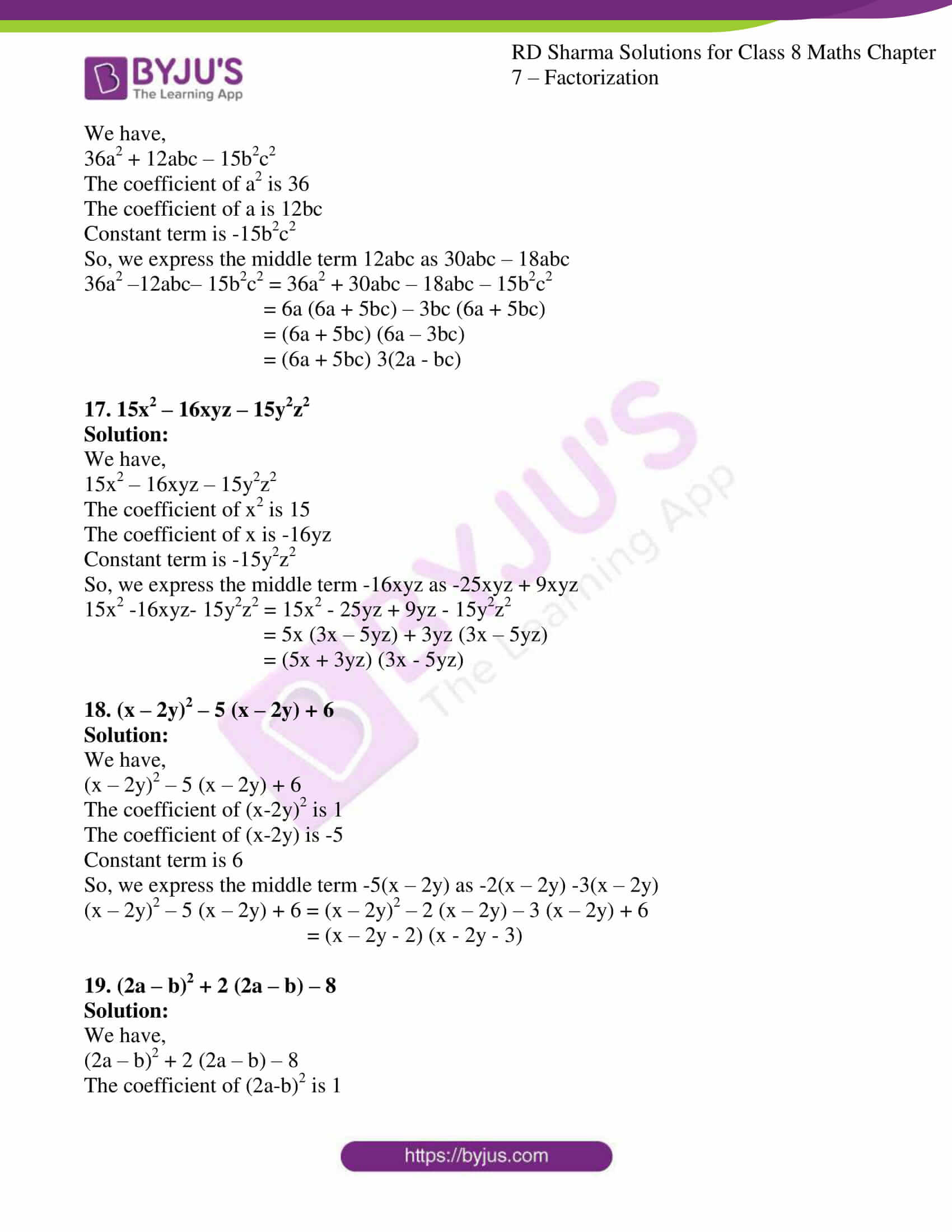### Access Answers to RD Sharma Solutions for Class 8 Maths Exercise 7.8 Chapter 7 Factorization

#### EXERCISE 7.8 PAGE NO: 7.30

Resolve each of the following quadratic trinomials into factors:

1. 2x2 + 5x + 3

Solution:

We have,

2x2 + 5x + 3

The coefficient of x2 is 2

The coefficient of x is 5

Constant term is 3

We shall split up the center term i.e., 5 into two parts such that their sum p+q is 5 and product pq = 2 × 3 is 6

So, we express the middle term 5x as 2x + 3x

2x2 + 5x + 3 = 2x2 + 2x + 3x + 3

= 2x (x + 1) + 3 (x + 1)

= (2x + 3) (x + 1)

2. 2x2 – 3x – 2

Solution:

We have,

2x2 – 3x – 2

The coefficient of x2 is 2

The coefficient of x is -3

Constant term is -2

So, we express the middle term -3x as -4x + x

2x2 – 3x – 2 = 2x2 – 4x + x – 2

= 2x (x – 2) + 1 (x – 2)

= (x – 2) (2x + 1)

3. 3x2 + 10x + 3

Solution:

We have,

3x2 + 10x + 3

The coefficient of x2 is 3

The coefficient of x is 10

Constant term is 3

So, we express the middle term 10x as 9x + x

3x2 + 10x + 3 = 3x2 + 9x + x + 3

= 3x (x + 3) + 1 (x + 3)

= (3x + 1) (x + 3)

4. 7x – 6 – 2x2

Solution:

We have,

7x – 6 – 2x2

– 2x2 + 7x – 6

2x2 – 7x + 6

The coefficient of x2 is 2

The coefficient of x is -7

Constant term is 6

So, we express the middle term -7x as -4x – 3x

2x2 – 7x + 6 = 2x2 – 4x – 3x + 6

= 2x (x – 2) – 3 (x – 2)

= (x – 2) (2x – 3)

5. 7x2 – 19x – 6

Solution:

We have,

7x2 – 19x – 6

The coefficient of x2 is 7

The coefficient of x is -19

Constant term is -6

So, we express the middle term -19x as 2x – 21x

7x2 – 19x – 6 = 7x2 + 2x – 21x – 6

= x (7x + 2) – 3 (7x + 2)

= (7x + 2) (x – 3)

6. 28 – 31x – 5x2

Solution:

We have,

28 – 31x – 5x2

– 5x2 -31x + 28

5x2 + 31x – 28

The coefficient of x2 is 5

The coefficient of x is 31

Constant term is -28

So, we express the middle term 31x as -4x + 35x

5x2 + 31x – 28 = 5x2 – 4x + 35x – 28

= x (5x – 4) + 7 (5x – 4)

= (x + 7) (5x – 4)

7. 3 + 23y – 8y2

Solution:

We have,

3 + 23y – 8y2

– 8y2 + 23y + 3

8y2 – 23y – 3

The coefficient of y2 is 8

The coefficient of y is -23

Constant term is -3

So, we express the middle term -23y as -24y + y

8y2 – 23y – 3 = 8y2 – 24y + y – 3

= 8y (y – 3) + 1 (y – 3)

= (8y + 1) (y – 3)

8. 11x2 – 54x + 63

Solution:

We have,

11x2 – 54x + 63

The coefficient of x2 is 11

The coefficient of x is -54

Constant term is 63

So, we express the middle term -54x as -33x – 21x

11x2 – 54x + 63 = 11x2 – 33x – 21x + 63

= 11x (x – 3) – 21 (x – 3)

= (11x – 21) (x – 3)

9. 7x – 6x2 + 20

Solution:

We have,

7x – 6x2 + 20

– 6x2 + 7x + 20

6x2 – 7x – 20

The coefficient of x2 is 6

The coefficient of x is -7

Constant term is -20

So, we express the middle term -7x as -15x + 8x

6x2 – 7x – 20 = 6x2 – 15x + 8x – 20

= 3x (2x – 5) + 4 (2x – 5)

= (3x + 4) (2x – 5)

10. 3x2 + 22x + 35

Solution:

We have,

3x2 + 22x + 35

The coefficient of x2 is 3

The coefficient of x is 22

Constant term is 35

So, we express the middle term 22x as 15x + 7x

3x2 + 22x + 35 = 3x2 + 15x + 7x + 35

= 3x (x + 5) + 7 (x + 5)

= (3x + 7) (x+ 5)

11. 12x2 – 17xy + 6y2

Solution:

We have,

12x2 – 17xy + 6y2

The coefficient of x2 is 12

The coefficient of x is -17y

Constant term is 6y2

So, we express the middle term -17xy as -9xy – 8xy

12x2 -17xy+ 6y2 = 12x2 – 9xy – 8xy + 6y2

= 3x (4x – 3y) – 2y (4x – 3y)

= (3x – 2y) (4x – 3y)

12. 6x2 – 5xy – 6y2

Solution:

We have,

6x2 – 5xy – 6y2

The coefficient of x2 is 6

The coefficient of x is -5y

Constant term is -6y2

So, we express the middle term -5xy as 4xy – 9xy

6x2 -5xy- 6y2 = 6x2 + 4xy – 9xy – 6y2

= 2x (3x + 2y) -3y (3x + 2y)

= (2x – 3y) (3x + 2y)

13. 6x2 – 13xy + 2y2

Solution:

We have,

6x2 – 13xy + 2y2

The coefficient of x2 is 6

The coefficient of x is -13y

Constant term is 2y2

So, we express the middle term -13xy as -12xy – xy

6x2 -13xy+ 2y2 = 6x2 – 12xy – xy + 2y2

= 6x (x – 2y) – y (x – 2y)

= (6x – y) (x – 2y)

14. 14x2 + 11xy – 15y2

Solution:

We have,

14x2 + 11xy – 15y2

The coefficient of x2 is 14

The coefficient of x is 11y

Constant term is -15y2

So, we express the middle term 11xy as 21xy – 10xy

14x2 + 11xy- 15y2 = 14x2 + 21xy – 10xy – 15y2

= 2x (7x – 5y) + 3y (7x – 5y)

= (2x + 3y) (7x – 5y)

15. 6a2 + 17ab – 3b2

Solution:

We have,

6a2 + 17ab – 3b2

The coefficient of a2 is 6

The coefficient of a is 17b

Constant term is -3b2

So, we express the middle term 17ab as 18ab – ab

6a2 +17ab– 3b2 = 6a2 + 18ab – ab – 3b2

= 6a (a + 3b) – b (a + 3b)

= (6a – b) (a + 3b)

16. 36a2 + 12abc – 15b2c2

Solution:

We have,

36a2 + 12abc – 15b2c2

The coefficient of a2 is 36

The coefficient of a is 12bc

Constant term is -15b2c2

So, we express the middle term 12abc as 30abc – 18abc

36a2 –12abc– 15b2c2 = 36a2 + 30abc – 18abc – 15b2c2

= 6a (6a + 5bc) – 3bc (6a + 5bc)

= (6a + 5bc) (6a – 3bc)

= (6a + 5bc) 3(2a – bc)

17. 15x2 – 16xyz – 15y2z2

Solution:

We have,

15x2 – 16xyz – 15y2z2

The coefficient of x2 is 15

The coefficient of x is -16yz

Constant term is -15y2z2

So, we express the middle term -16xyz as -25xyz + 9xyz

15x2 -16xyz- 15y2z2 = 15x2 – 25yz + 9yz – 15y2z2

= 5x (3x – 5yz) + 3yz (3x – 5yz)

= (5x + 3yz) (3x – 5yz)

18. (x – 2y)2 – 5 (x – 2y) + 6

Solution:

We have,

(x – 2y)2 – 5 (x – 2y) + 6

The coefficient of (x-2y)2 is 1

The coefficient of (x-2y) is -5

Constant term is 6

So, we express the middle term -5(x – 2y) as -2(x – 2y) -3(x – 2y)

(x – 2y)2 – 5 (x – 2y) + 6 = (x – 2y)2 – 2 (x – 2y) – 3 (x – 2y) + 6

= (x – 2y – 2) (x – 2y – 3)

19. (2a – b)2 + 2 (2a – b) – 8

Solution:

We have,

(2a – b)2 + 2 (2a – b) – 8

The coefficient of (2a-b)2 is 1

The coefficient of (2a-b) is 2

Constant term is -8

So, we express the middle term 2(2a – b) as 4 (2a –b) – 2 (2a – b)

(2a – b)2 + 2 (2a – b) – 8 = (2a – b)2 + 4 (2a – b) – 2 (2a – b) – 8

= (2a – b) (2a – b + 4) – 2 (2a – b + 4)

= (2a – b + 4) (2a – b – 2)